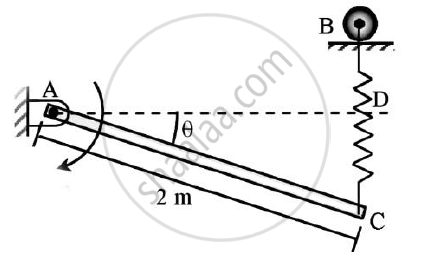# Determine the Required Stiffness K So that the Uniform 7 Kg Bar Ac is in Equilibrium When θ=30o. - Engineering Mechanics

Determine the required stiffness k so that the uniform 7 kg bar AC is in equilibrium when θ=30o.
Due to the collar guide at B the spring remains vertical and is unstretched when θ = 0o.Use principle of virtual work.#### Solution

Solution:
Given : : Mass of bar AC = 7 kg
θ = 30°
To find : Required stiffness k
Solution:
Weight of rod = 7g N
Assume rod AC have a small virtual angular displacement 𝛿θ in anti-clockwise direction
Reaction forces HA and VA do not do any virtual work
Un-stretched length of the spring = BD
Extension of the spring (x) = CD = 2sin θ
Assume FS be the spring force at end C of the rod
FS = Kx = 2Ksin θ
Assume A to be the origin and AD be the X-axis of the system

 Active force Co-ordinate of the point of action along the force Virtual Displacement W=7g -sin θ 𝛿yM=-cos θ 𝛿 θ FS=2Ksin θ -2sin θ 𝛿yC’=-2cos θ 𝛿 θ

APPLYING PRINCIPLE OF VIRTUAL WORK
𝛿U = 0
-W X 𝛿YM + FS X 𝛿YC’ + 50 X 𝛿 θ = 0
2Ksin θ x (-2cos θ 𝛿 θ) = 7g x (-cos θ 𝛿 θ) - 50 x 𝛿 θ
Substituting the value of θ and solving
K=63.2025 Nm
The required stiffness K for bar AC to remain in equilibrium is 63.2025 Nm

Concept: Applications on Equilibrium Mechanisms
Is there an error in this question or solution?